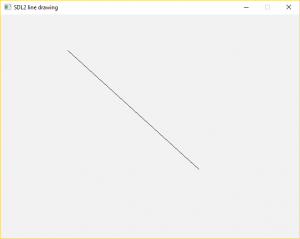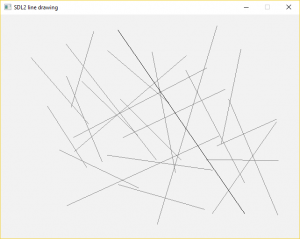# Drawing Lines with SDL2

We’ve seen before how we can draw pixels using SDL2, similar to a basic Paint application. The next step is to draw lines. To do this, we can reuse our pixel drawing code with something like Bresenham’s line algorithm, or we can use SDL_RenderDrawLine(), which SDL2 gives us out of the box.

Let’s start with the following code, which gives us a basic empty window:

```#include <SDL.h>

int main(int argc, char ** argv)
{
// variables

bool quit = false;
SDL_Event event;

// init SDL

SDL_Init(SDL_INIT_VIDEO);
SDL_Window * window = SDL_CreateWindow("SDL2 line drawing",
SDL_WINDOWPOS_UNDEFINED, SDL_WINDOWPOS_UNDEFINED, 640, 480, 0);
SDL_Renderer * renderer = SDL_CreateRenderer(window, -1, 0);

// handle events

while (!quit)
{
SDL_Delay(10);
SDL_PollEvent(&event);

switch (event.type)
{
case SDL_QUIT:
quit = true;
break;
// TODO input handling code goes here
}

// clear window

SDL_SetRenderDrawColor(renderer, 242, 242, 242, 255);
SDL_RenderClear(renderer);

// TODO rendering code goes here

// render window

SDL_RenderPresent(renderer);
}

// cleanup SDL

SDL_DestroyRenderer(renderer);
SDL_DestroyWindow(window);
SDL_Quit();

return 0;
}
```

A line is usually drawn between two endpoints: (x1, y1) and (x2, y2). When the user presses the left mouse button, we’ll store the place he clicked as (x1, y1), and then draw a line to the current cursor position (x2, y2). When he lets go of the left mouse button, we’ll store that line.

Thus, we need an integer variable for each coordinate, and a boolean that indicates whether a line is being drawn (i.e. whether the left mouse button is pressed):

```    int x1 = 0;
int y1 = 0;
int x2 = 0;
int y2 = 0;
bool drawing = false;
```

We can now add handlers for when the left mouse button is pressed and released. At a basic level, this involves toggling the `drawing` flag. However, when the left mouse button is pressed (i.e. when a new line is started), we also need to record the current cursor position as (x1, y1), and reset the value of (x2, y2).

```            case SDL_MOUSEBUTTONDOWN:
switch (event.button.button)
{
case SDL_BUTTON_LEFT:
x1 = event.motion.x;
y1 = event.motion.y;
x2 = event.motion.x;
y2 = event.motion.y;
drawing = true;
break;
}
break;
case SDL_MOUSEBUTTONUP:
switch (event.button.button)
{
case SDL_BUTTON_LEFT:
drawing = false;
break;
}
break;
```

An additional event handler, this time for mouse movement, updates the value of (x2, y2) while the left mouse button is pressed, giving a feel that the line is being dragged:

```            case SDL_MOUSEMOTION:
if (drawing)
{
x2 = event.motion.x;
y2 = event.motion.y;
}
break;
```

We can now add code to actually draw the line, before the call to `SDL_RenderPresent()`:

```        // draw current line

SDL_SetRenderDrawColor(renderer, 0, 0, 0, 255);
if (drawing)
SDL_RenderDrawLine(renderer, x1, y1, x2, y2);
```

Great, we now can draw lines by left-clicking and dragging the mouse:…but the lines disappear whenever the left mouse button is released!

In order to keep the drawn lines in the window, we’ll need to store them, and draw them on each iteration of the game loop. We first need to declare a `struct` that represents each line we draw:

```struct Line
{
int x1;
int y1;
int x2;
int y2;
};
```

We then need a simple data structure to store the lines, such as a list from the C++ standard library:

```#include <list>
```

Let’s declare a variable for the list that will hold our lines:

```std::list<Line> lines;
```

Then, we’ll add some code so that when the left mouse button is released, the new line is added to the list:

```            case SDL_MOUSEBUTTONUP:
switch (event.button.button)
{
case SDL_BUTTON_LEFT:
drawing = false;
Line line = { x1, y1, x2, y2 };
lines.push_back(line);
break;
}
break;
```

Finally, before the code that draws the new line, let’s add some code that draws all the old ones:

```        // draw stored lines

SDL_SetRenderDrawColor(renderer, 128, 128, 128, 255);

for (std::list<Line>::const_iterator i = lines.begin(); i != lines.end(); ++i)
{
Line line = *i;
SDL_RenderDrawLine(renderer, line.x1, line.y1, line.x2, line.y2);
}
```

Now, all the lines you draw will remain in the window: